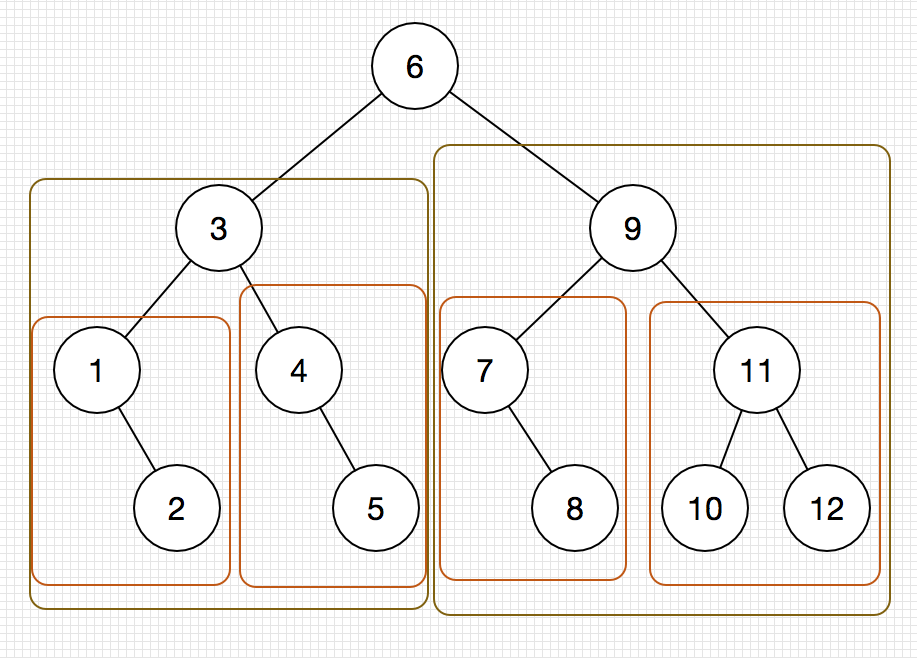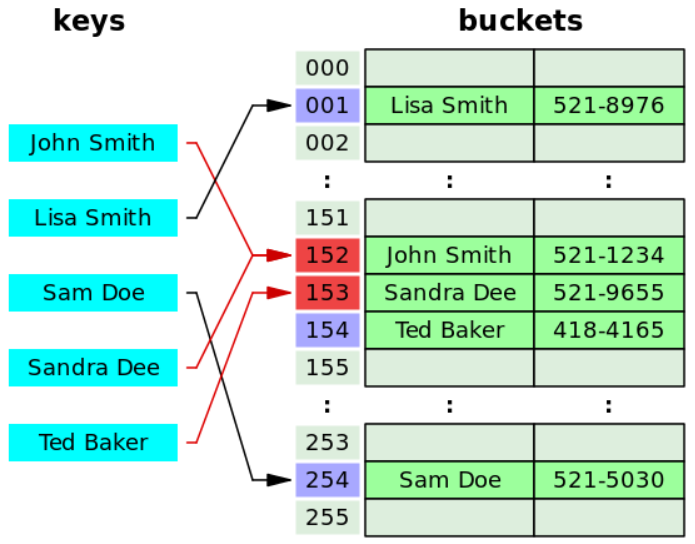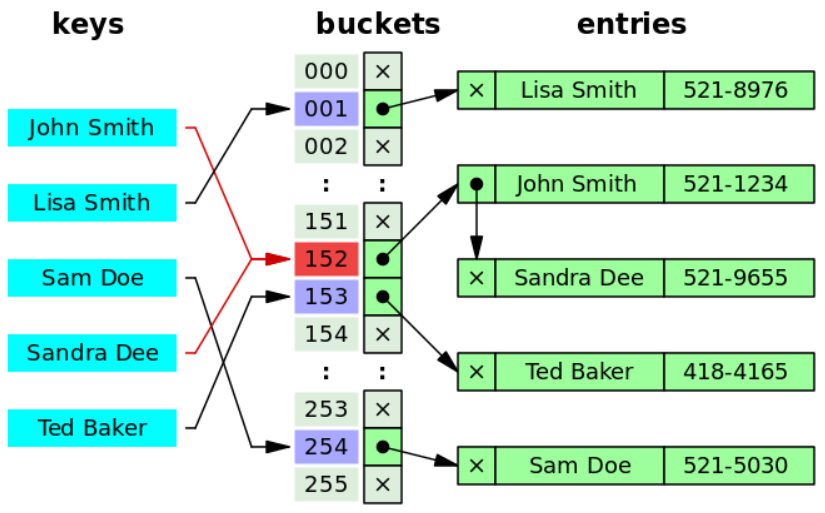# 0x00 查找

• 顺序查找（Linear Search）
• 二分查找（又称折半查找Binary Search）

• 哈希表（又称散列表Hash Table）
• B树（参见树那一节）

ASL的计算公式如下：
$ASL=\sum_{i=1}^n P_i\times C_i$

• n为查找长度
• $P_i$
为第
$i$
个元素为目标的概率
• $C_i$
为找到第
$i$
个元素所需要进行的比较次数

# 0x01 顺序查找和二分查找

## 0x00 顺序查找

$ASL=\sum_{i=1}^n \frac{i}{n} = \frac{n+1}{2}$

$\frac{1}{n}$
，当定位目标元素为第
$i$

$i$

$n+1$
，平均查找长度即为
$ASL=n+1$

1
1 2 4 5 6 7 8 9
Copied!

$ASL_{查找失败时}=\frac{1+2+3+...+n+n}{n+1}=\frac{n}{2}+\frac{n}{n+1}$

$\frac{1}{n+1}$
，此处n+1的原因是有n+1个查找失败的顶点，因为我把整个表全部扫描了一遍，我最后还多了一次判断输入是否到头的比较过程。分子最后多了个+n的原因是，当查找进行到输入数据末尾时，由于每一次查找都多了一次比较当前元素是否小于被查找元素的过程，有n个元素，自然也多了n次比较小于的过程。

## 0x01 二分查找

1
class Solution {
2
public:
3
int search(std::vector<int>& nums, int target) {
4
int lo = 0, hi = nums.size(), mid;
5
while (lo < hi)
6
{
7
mid = (lo + hi) >> 1;
8
if (nums[mid] == target)
9
{
10
return mid;
11
}
12
else if(nums[mid] > target)
13
{
14
hi = mid;
15
}
16
else
17
{
18
lo = mid + 1;
19
}
20
}
21
return -1;
22
}
23
};
Copied!

1
1 2 3 4 5 6 7 8 9 10 11 12
Copied!$ASL=\sum_{i=1}^h{\frac{1}{n}\times (i\times2^{i-1})} \\ ASL=\frac{1}{n} \times (1\times1+2\times2+...+h\times2^{h-1})\\ ASL=\frac{n+1}{n}\log_2{(n+1)}-1 \\ \Rightarrow ASL\approx \log_2{(n+1)}-1$

$h=\lceil \log_2{(N+1)} \rceil$

$O(\log_2{N})$

## 0x02 分块查找

• 将查找表分为若干块，块内的元素可以是无序的，但是块与块之间是有序的，例如第一个块中的最大关键字小于第二个块中的最小关键字，以此类推。
• 有一个索引表，索引表包含了每个块含有的最大关键字，以及第一个起始元素的位置，索引表按关键字有序排列。

$L_I$
，块内查找的平均查找长度记为
$L_S$
，则ASL有如下计算：
$ASL=L_I+L_S\\$

$ASL=\lceil \log_2{(b+1)} \rceil+\frac{s+1}{2}$

$ASL=\frac{b+1}{2}+\frac{s+1}{2}\\ 代入b=\frac{n}{s}有:ASL=\frac{n+s}{2s}+\frac{s+1}{2}\\ \Rightarrow ASL=\frac{s^2+2s+n}{2s}$

# 0x02 哈希表

## 0x00 基本概念

• 散列函数（Hash function）：用于把查找表中的关键字映射成该关键字对应地址的函数，国内教材上一般记为：
1
Copied!
国外教材上一般写为如下两种形式：
1
// 通过散列函数f得到关键字key的地址index
2
// 其中散列函数需传入查找表数组的大小array_size
3
index = f(key, array_size);
Copied!
或者：
1
// 通过散列函数hashfunc得到关键字key所对应的地址hash
2
hash = hashfunc(key);
3
// 通过这个地址hash与查找表数组大小进行模运算以获取其在查找表的索引地址
4
index = hash % array_size;
Copied!
• 散列表：根据关键字直接访问的数据结构，其建立了关键字和存储地址之间的一种直接映射关系

$O(1)$

$\text{load factor}=\frac{\text{size}}{\text{capacity}}$

## 0x01 散列函数的构造

• 散列函数的定义域必需包含全部需要存储的关键字，而值域的范围则依赖于散列表的大小或地址范围
• 散列函数计算出来的地址应该能等概率地、离散地、均匀地分布在整个地址空间（discrete uniform distribution），从而减少冲突的发生
• 散列函数应尽量简单，在较短的时间复杂度内算出任一关键字所对应的散列地址

### 0x00 直接定址法

1
2
return a*key + b; // 此处的a和b为常数
3
}
Copied!

### 0x01 除留余数法

1
2
return key % p; // 其中p为质数
3
}
Copied!

## 0x02 冲突处理

### 0x00 开放定址法

$Addr_i = (\text{Hashfunc}(key)+d_i)\%m$

$d_i$

$Addr_i$

• 线性探测法（Linear probing）：冲突发生时，顺序查看表中的下一个单元，直到找到一个空闲单元把他放进去，如果遇到表尾，那就从表首地址处继续，所以，在表未满的情况下，总能找到一个空闲单元。
线性探测法可能使第n个散列地址的同义词存入n+1的位置，然后本该存储n+1位置的元素又要去争抢n+2的位置，以此类推，从而会造成大的元素在相邻的散列地址上聚集起来（Primary clustering），降低了查找效率。如下图：$H+1, H+2,...,H+k$
如果使用平方探测法，接下来的判定序列即为：
$H+1^2, H+2^2,...,H+k^2$
其中
$k \leqslant \frac{m}{2}$
，且散列表的长度m必需是一个可表示成4k+3的素数。 别看仅仅在线性探测的序列上对增量加了个平方，这却有效地缓解了线性探测中失效后元素的聚集问题（clustering problem）。 它的缺点在于不能探测到散列表上的所有单元，但至少能探测到一半的单元。 这种方法被用于Unix文件系统（Unix File System又称Berkeley Fast File System）用于分配新的空闲块
• 再散列法（Double hashing）：此种方法需要使用2个散列函数，当第一个散列函数计算出来的地址值发生冲突时，再由第二个散列函数计算该关键字的地址增量。其具体的散列函数形式如下：
$Addr_i = (\text{Hash}_1(key)+i\times \text{Hash}_2(key))\%m$
上式中的
$i$
为冲突发生的次数，初始为0，再散列法中，最多经过m-1次探测就会遍历表中所有的位置回到
$Addr_0$
的位置

### 0x01 拉链法## 0x03 性能分析

• 首先通过散列函数通过查找的关键字计算出其散列地址
• 然后查找表中相应地址是否存在元素，若没有则查找失败，若有则比较其与被查找的值是否相同，若相同则返回查找成功的标识，若不相同则执行下一个步骤
• 使用哈希表给定的冲突处理的方法来计算下一个散列地址，然后转入上一个步骤继续执行

$O(1+\text{load factor})=O(1)$
，在最坏情况下，采用拉链法处理冲突时装填因子是有可能大于1的，这样时间就消耗在了遍历这个链表上，此时的时间复杂度为
$O(N)$
。同样采用开放定址法处理冲突，当hash函数始终返回一个固定的地址值，但是这个地址值存储的元素又不为所需查找的元素时，也需要依次地向下遍历直到找到元素，最坏的情况下，遍历一周再回到hash地址值前一个，所需的最坏时间复杂度仍为
$O(N)$

# 0x03 字符串的模式匹配

## 0x00 暴力破解

1
class Solution {
2
public:
3
int strStr(std::string haystack, std::string needle) {
4
if (needle.size() == 0)
5
{
6
return 0;
7
}
8
if (haystack.size() >= needle.size())
9
{
10
for (int i = 0; i < haystack.size() - needle.size() + 1; ++i)
11
{
12
if (haystack[i] == needle)
13
{
14
int j = 0;
15
for (; j < needle.size(); ++j)
16
{
17
if (haystack[i + j] != needle[j])
18
{
19
break;
20
}
21
}
22
if (j == needle.size())
23
{
24
return i;
25
}
26
}
27
}
28
}
29
return -1;
30
}
31
};
Copied!

Clarification:
What should we return when needle is an empty string? This is a great question to ask during an interview.
For the purpose of this problem, we will return 0 when needle is an empty string. This is consistent to C's strstr() and Java's [indexOf()](https://docs.oracle.com/javase/7/docs/api/java/lang/String.html#indexOf(java.lang.String)).

$O(N\times M)$
，其中的
$N$
$M$

$O(\min{(N,M)}^2) \leqslant O(N\times M)$

## 0x01 KMP算法

KMP算法（KMP algorithm）的全称是三个人的名字，是一种改进后的字符串模式匹配算法，可以在
$O(N+M)$

For the past few days, I’ve been reading various explanations of the Knuth-Morris-Pratt string searching algorithms. For some reason, none of the explanations were doing it for me. I kept banging my head against a brick wall once I started reading “the prefix of the suffix of the prefix of the…”.

1
S: bacbababaabcbab
2
W: abababca
Copied!

1
char: | a | b | a | b | a | b | c | a |
2
index: | 0 | 1 | 2 | 3 | 4 | 5 | 6 | 7 |
3
value: | 0 | 0 | 1 | 2 | 3 | 4 | 0 | 1 |
Copied!

1
S: bacbababaabcbab
2
|-
3
W: abababca
Copied!

1
S: bacbababaabcbab
2
W: abababca
Copied!
S中的c和W中的a并不匹配，再往下走一个，依次重复此过程，直到走到了a：
1
S: bacbababaabcbab
2
|||||-
3
W: abababca
Copied!

1

Copied!

1
S: bacbababaabcbab
2
xx|||-
3
W: abababca
Copied!

1
S: bacbababaabcbab
2
xx|- -
3
W: abababca
Copied!

• Proper prefix（前缀）：就是除了最后一个字符外，一个字符串的所有头部组合（cut off），就比如说Snape，它的Proper prefix就有S, Sn, Sna, Snap这样。
• Proper suffix（后缀）：就是除去第一个字符外，一个字符串全部的尾部的组合（cut off），就比如说单词agrid，它的Proper suffix就有grid, rid, id, d这样。
TL;DR

• a的前缀和后缀均为空，共有的元素长度为0
• ab的前缀为a，后缀为b，共有的元素长度为0
• aba的前缀为a, ab，后缀为ba, a，两者共有的元素仅有a长度为1
• abab的前缀为a, ab, aba，后缀为bab, ab, b，两者共有的元素仅有ab其长度为2
• ababa的前缀为a, ab, aba, abab，后缀为baba, aba, ba, a，两者共有的元素有abaa，最长公共元素为aba，长度为3
• ababab的前缀为a, ab, aba, abab, ababa，后缀为babab, abab, bab, ab, b，最长公共元素为abab，长度为4
• abababc的前缀为a, ab, aba, abab, ababa, ababab，后缀为bababc, ababc, babc, abc, bc, c，没有一个相同的，共有的元素长度为0
• abababca的前缀为a, ab, aba, abab, ababa, ababab, abababc，后缀为bababca, ababca, babca, abca, bca, ca, a，最长公共元素为a，长度为1

1
char: | a | b | a | b | a | b | c | a |
2
index: | 0 | 1 | 2 | 3 | 4 | 5 | 6 | 7 |
3
value: | 0 | 0 | 1 | 2 | 3 | 4 | 0 | 1 |
Copied!

KMP算法的时间复杂度为
$O(N+M)$
，但是在一般情况下，使用暴力破解的时间复杂度也基本近似
$O(N+M)$
，这也是为什么在LeetCode题目28. Implement strStr()下使用暴力破解和KMP算法求解的运行时间都可以超过100%的人的原因。KMP算法往往适用于主串与子串有很多部分匹配的时候，这样才能显得KMP算法比暴力破解快很多，因其主要的优点就是主串不回溯。

next数组其实还有一种求法，就是模式串对自身进行匹配运算，在任一位置往前能够匹配的最大长度即为当前的next数组的值，还是给定模式串abababca记为S，我们从next看起，模式串对自身进行匹配运算，此时b和a不相等，能匹配的最长长度为0，所以next=0
1
i
2
|
3
abababca next = 0
4
abababca
5
|
6
j
Copied!

1
i
2
|
3
abababca next = 1
4
abababca
5
|
6
j
Copied!

1
i
2
|
3
abababca next = 2
4
abababca
5
|
6
j
Copied!

1
i
2
|
3
abababca next = 3
4
abababca
5
|
6
j
Copied!
1
i
2
|
3
abababca next = 4
4
abababca
5
|
6
j
Copied!

1
i
2
|
3
abababca
4
abababca
5
|
6
j
Copied!

1
i
2
|
3
abababca next = 0
4
abababca
5
|
6
j
Copied!

1
i
2
|
3
abababca next = 1
4
abababca
5
|
6
j
Copied!

1
void getPartialMatchTable(std::string& needle,
2
std::vector<int>& next)
3
{
4
int i = 0;
5
int j = -1;
6
next = -1;
7
while (i < needle.size() - 1)
8
{
9
if (j == -1 || needle[i] == needle[j])
10
{
11
next[++i] = ++j;
12
}
13
else
14
{
15
j = next[j];
16
}
17
}
18
}
Copied!

1
class Solution {
2
3
void getPartialMatchTable(std::string& needle, std::vector<int>& next)
4
{
5
int i = 0;
6
int j = -1;
7
next = -1;
8
while (i < needle.size() - 1)
9
{
10
if (j == -1 || needle[i] == needle[j])
11
{
12
next[++i] = ++j;
13
}
14
else
15
{
16
j = next[j];
17
}
18
}
19
}
20
public:
21
int strStr(std::string haystack, std::string needle) {
22
if (needle.size() == 0)
23
{
24
return 0;
25
}
26
std::vector<int> next(needle.size());
27
getPartialMatchTable(needle, next);
28
29
int i = 0;
30
int j = 0;
31
int hs = haystack.size();
32
int ns = needle.size();
33
34
while (i < hs && j < ns)
35
{
36
if (j == -1 || haystack[i] == needle[j])
37
{
38
++i;
39
++j;
40
}
41
else
42
{
43
j = next[j];
44
}
45
}
46
return j == needle.size() ? i - j : -1;
47
}
48
};
Copied!

1
while (i < haystack.size() && j < needle.size())
2
... omitted ...
Copied!

1
int hs = haystack.size();
2
int ns = needle.size();
3
while (i < hs && j < ns)
4
... omitted ...
Copied!

1
Warning C4018 '<': signed/unsigned mismatch
Copied!

1
int(-1) < unsigned(5)
Copied!